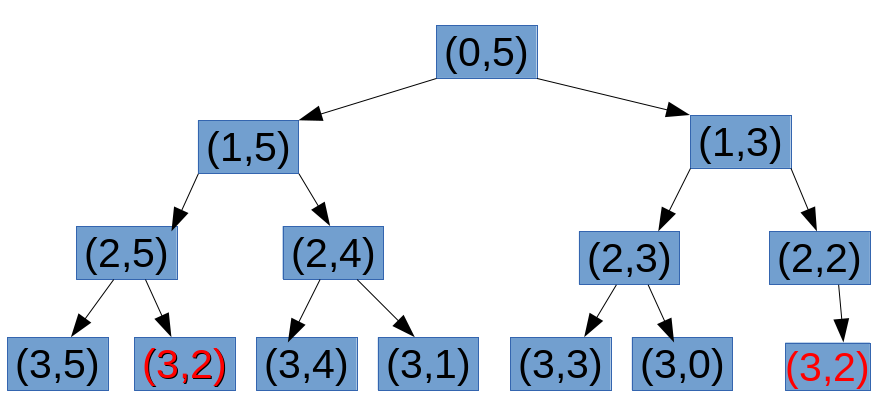# 动态规划分析总结——如何设计和实现动态规划算法

动态规划第一讲——缓存与动态规划

int  solution(int n){
if(n>0 && n<2) return n;
return solution(n-1) + solution(n-2);
}

如果我们计算F(10), 先需要计算F(9) F(8); 但是我们计算F(9)的时候，又需要计算F(8)，很明显，F(8)被计算了多次，存在重复计算；同理F(3)被重复计算的次数就更多了。算法分析与设计的核心在于 根据题目特点，减少重复计算。  在不改变算法结构的情况下，我们可以做如下改进：
int dp;
int  solution(int n){
if(n>0 && n<2) return n;
if(dp[n]!=0) return dp[n];
dp[n] = solution(n-1) + solution(n-2);
return  dp[n];
}

int  solution(int n){
int dp[n+1];
dp=1;dp=2;
for (i = 3; i <= n; ++i){
dp[n] = dp[n-1] + dp[n-2];
}
return  dp[n];
}

## 例二：01背包问题

weight=2, 1, 3, 2
value =3, 2, 4, 2
W=5

int rec(int i, int j){//从i到n号物品，选择重量不大于j的物品的最大价值
int res;
if(i==n){
res=0;
}
else if(j< w[i]){
res = rec(i+1, j);
}
else{
res = max(rec(i+1, j), rec(i+1, j-w[i])+v[i]);
}
return res;
}dp[i][j] = max(dp[i+1][j], dp[i+1][j-w[i]] + v[i]);//对应的计算表格如下和程序如下：
void solution(){
fill(dp[n], dp[n]+W, 0);
for (int i = n-1; i >= 0; --i){
for (j = 0; j <= W; ++j){
if(j < w[i]) dp[i][j] = dp[i+1][j];
else dp[i][j] = max(dp[i+1][j], dp[i+!][j-w[i]]+v[i]);
}
}
return dp[W];
}

dp[i][j] = max(dp[i-1][j] , dp[i-1][j-w[i]] + v[i]);

1>DP算法起源于DC—— 一个问题的解，可以先分解为求解一系列子问题的解，同时包含重叠子问题：于是，我们得到DP算法的第一个黄金准则：某个问题具有独立而重叠的字问题；子问题不独立，没法进行分治；子问题不重叠，没有进行DP的必要，直接用普通的分治法就可以了。
2>DP算法黄金准则2： 最优子问题—— 子问题的最优解可以推出原问题的最优解。

©️2019 CSDN 皮肤主题: 大白 设计师: CSDN官方博客